### FACTOR Statement

FACTOR <EFA-options |CFA-spec> ;

where EFA-options are options for the exploratory factor analysis that are described in the section Exploratory Factor Analysis and CFA-spec is a specification of confirmatory factor analysis that is described in the section Confirmatory Factor Analysis.

In the FACTOR statement, you can specify either EFA-options, CFA-spec, or neither of these. However, you cannot specify both EFA-options and CFA-spec at the same time. If no option is specified or there is at least one EFA-option (exploratory factor analysis option) specified in the FACTOR statement, an exploratory factor model is analyzed. Otherwise, a confirmatory factor model is analyzed with the CFA-spec. These two types of models are discussed in the next two sections.

#### Exploratory Factor Analysis

FACTOR <EFA-options> ;

For the exploratory factor model with orthogonal factors, PROC CALIS assumes the following model structures for the population covariance or correlation matrix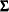: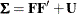where
is the factor loading matrix and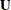is a diagonal matrix of error variances. In this section, p denotes the number of manifest variables corresponding to the rows and columns of matrix, and n denotes the number of factors (or components, if the COMPONENT option is specified in the FACTOR statement) corresponding to the columns of the factor loading matrix. While the number of manifest variables is set automatically by the number of variables in the VAR statement or in the input data set, the number of factors can be set by the N= option in the FACTOR statement.

The unrestricted exploratory factor model is not identified because any orthogonal rotated factor loading matrix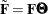satisfies the same model structures asdoes, where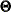is any orthogonal matrix so that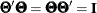. Mathematically, the covariance or correlation structures can be expressed as: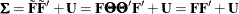To obtain an identified orthogonal factor solution as a starting point, the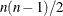elements in the upper triangle ofare constrained to zeros in PROC CALIS. Initial estimates for factor loadings and unique variances are computed by an algebraic method of approximate factor analysis. Given the initial estimates, final estimates are obtained through the iterative optimization of an objective function, which depends on the estimation method specified in the METHOD= option (default with ML—maximum likelihood) of the PROC CALIS statement.

To make the factor solution more interpretable, you can use the ROTATE= option in the FACTOR statement to obtain a rotated factor loading matrix with a simple pattern. Rotation can be orthogonal or oblique. The rotated factors remain uncorrelated after an orthogonal rotation but would be correlated after an oblique rotation. The model structures of an oblique solution are expressed in the following equation: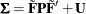where
is the rotated factor loading matrix and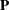is a symmetric matrix for factor correlations. See the sections The FACTOR Model and Exploratory Factor Analysis Models for more details about exploratory factor models.

You can also do exploratory factor analysis by the more dedicated FACTOR procedure. Even though extensive comparisons of the factor analysis capabilities between the FACTOR and CALIS procedures are not attempted here, some general points can be made here. In general, the FACTOR procedure provides more factor analysis options than the CALIS procedure does, although both procedures have some unique factor analysis features that are not shared by the other. PROC CALIS requires more computing time and memory than PROC FACTOR because it is designed for more general structural estimation problems and is not able to exploit all the special properties of the unconstrained factor analysis model. For maximum likelihood analysis, you can use either PROC FACTOR (with METHOD=ML, which is not the default method in PROC FACTOR) or PROC CALIS. Because the initial unrotated factor solution obtained by PROC FACTOR uses a different set of identification constraints than that of PROC CALIS, you would observe different initial ML factor solutions for the procedures. Nonetheless, the initial solutions by both procedures are statistically equivalent.

The following EFA-options are available in the FACTOR statement:

COMPONENT | COMP

computes a component analysis instead of a factor analysis (the diagonal matrixin the model is set to 0). Note that the rank of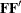is equal to the number n of components in. If n is smaller than the number of variables in the moment matrix, the matrix of predicted model values is singular and maximum likelihood estimates forcannot be computed. You should compute ULS estimates in this case.

HEYWOOD | HEY

constrains the diagonal elements ofto be nonnegative. Equivalently, you can constrain these elements to positive values by the BOUNDS statement.

GAMMA=p

specifies the orthomax weight used with the option ROTATE=ORTHOMAX. Alternatively, you can use ROTATE=ORTHOMAX(p) with p representing the orthomax weight. There is no restriction on valid values for the orthomax weight, although the most common values are between 0 and the number of variables. The default GAMMA= value is one, resulting in the varimax rotation.

N=n

specifies the number of first-order factors or components. The number of factors (n) should not exceed the number of manifest variables (p) in the analysis. For the saturated model with n = p, the COMP option should generally be specified for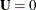; otherwise,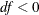. For n = 0 no factor loadings are estimated, and the model is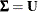, with a diagonalmatrix. By default, n = 1.

NORM< = KAISER  |  NONE>

Kaiser-normalizes the rows of the factor pattern for rotation. NORM=KAISER, which is the default, has exactly the same effect as NORM. You can turn off the normalization by NORM=NONE.

RCONVERGE=p
RCONV=p

specifies the convergence criterion for rotation cycles. Rotation stops when the scaled change of the simplicity function value is less than the RCONVERGE= value. The default convergence criterion is: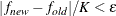where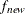and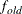are simplicity function values of the current cycle and the previous cycle, respectively,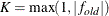is a scaling factor, andis 1E–9 by default and is modified by the RCONVERGE= value.

RITER=i

specifies the maximum number of cycles i for factor rotation. The default i is the greater of 10 times the number of variables and 100.

ROTATE | R=name

specifies an orthogonal or oblique rotation of the initial factor solution. Although ROTATE=PRINCIPAL is actually not a rotation method, it is put here for convenience. By default, ROTATE=NONE.

Valid names for orthogonal rotations are as follows:

BIQUARTIMAX | BIQMAX

specifies orthogonal biquartimax rotation. This corresponds to the specification ROTATE=ORTHOMAX(0.5).

EQUAMAX | E

specifies orthogonal equamax rotation. This corresponds to the specification ROTATE=ORTHOMAX with GAMMA=n/2.

FACTORPARSIMAX | FPA

specifies orthogonal factor parsimax rotation. This corresponds to the specification ROTATE=ORTHOMAX with GAMMA=n.

NONE | N

specifies that no rotation be performed, leaving the original orthogonal solution.

ORTHCF(p1,p2) |  ORCF(p1,p2)

specifies the orthogonal Crawford-Ferguson rotation (Crawford and Ferguson, 1970) with the weights p1 and p2 for variable parsimony and factor parsimony, respectively. See the definitions of weights in Chapter 35: The FACTOR Procedure.

ORTHGENCF(p1,p2,p3,p4) |  ORGENCF(p1,p2,p3,p4)

specifies the orthogonal generalized Crawford-Ferguson rotation (Jennrich, 1973), with the four weights p1, p2, p3, and p4. For the definitions of these weights, see the section Simplicity Functions for Rotations in Chapter 35: The FACTOR Procedure.

ORTHOMAX<(p1)> | ORMAX<(p1)>

specifies the orthomax rotation (see Harman 1976) with orthomax weight p1. If ROTATE=ORTHOMAX is used, the default p1 value is 1 unless specified otherwise in the GAMMA= option. Alternatively, ROTATE=ORTHOMAX(p1) specifies p1 as the orthomax weight or the GAMMA= value. For the definitions of the orthomax weight, see the section Simplicity Functions for Rotations in Chapter 35: The FACTOR Procedure.

PARSIMAX | PA

specifies orthogonal parsimax rotation. This corresponds to the specification ROTATE=ORTHOMAX with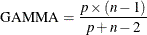PRINCIPAL | PC

specifies a principal axis rotation. If ROTATE=PRINCIPAL is used with a factor rather than a component model, the following rotation is performed: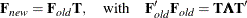where the columns of matrix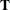contain the eigenvectors of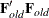.

QUARTIMAX | QMAX | Q

specifies orthogonal quartimax rotation. This corresponds to the specification ROTATE=ORTHOMAX(0).

VARIMAX | V

specifies orthogonal varimax rotation. This corresponds to the specification ROTATE=ORTHOMAX with GAMMA=1.

Valid names for oblique rotations are as follows:

BIQUARTIMIN | BIQMIN

specifies biquartimin rotation. It corresponds to the specification ROTATE=OBLIMIN(.5) or ROTATE=OBLIMIN with TAU=.5.

COVARIMIN | CVMIN

specifies covarimin rotation. It corresponds to the specification ROTATE=OBLIMIN(1) or ROTATE=OBLIMIN with TAU=1.

OBBIQUARTIMAX | OBIQMAX

specifies oblique biquartimax rotation.

OBEQUAMAX | OE

specifies oblique equamax rotation.

OBFACTORPARSIMAX | OFPA

specifies oblique factor parsimax rotation.

OBLICF(p1,p2) |  OBCF(p1,p2)

specifies the oblique Crawford-Ferguson rotation (Crawford and Ferguson, 1970) with the weights p1 and p2 for variable parsimony and factor parsimony, respectively. For the definitions of these weights, see the section Simplicity Functions for Rotations in Chapter 35: The FACTOR Procedure.

OBLIGENCF(p1,p2,p3,p4) |  OBGENCF(p1,p2,p3,p4)

specifies the oblique generalized Crawford-Ferguson rotation (Jennrich, 1973) with the four weights p1, p2, p3, and p4. For the definitions of these weights, see the section Simplicity Functions for Rotations in Chapter 35: The FACTOR Procedure.

OBLIMIN<(p1)> | OBMIN<(p1)>

specifies the oblimin rotation with oblimin weight p1. If ROTATE=OBLIMIN is used, the default p1 value is zero unless specified otherwise in the TAU= option. Alternatively, ROTATE=OBLIMIN(p1) specifies p1 as the oblimin weight or the TAU= value. For the definitions of the oblimin weight, see the section Simplicity Functions for Rotations in Chapter 35: The FACTOR Procedure.

OBPARSIMAX | OPA

specifies oblique parsimax rotation.

OBQUARTIMAX | OQMAX

specifies oblique quartimax rotation. This is the same as the QUARTIMIN method.

OBVARIMAX | OV

specifies oblique varimax rotation.

QUARTIMIN | QMIN

specifies quartimin rotation. It is the same as the oblique quartimax method. It also corresponds to the specification ROTATE=OBLIMIN(0) or ROTATE=OBLIMIN with TAU=0.

TAU=p

specifies the oblimin weight used with the option ROTATE=OBLIMIN. Alternatively, you can use ROTATE=OBLIMIN(p) with p representing the oblimin weight. There is no restriction on valid values for the oblimin weight, although for practical purposes a negative or zero value is recommended. The default TAU= value is 0, resulting in the quartimin rotation.

#### Confirmatory Factor Analysis

FACTOR factor-variables-relation <, factor-variables-relation …> ;

where each factor-variables-relation is defined as factor right-arrow var-list < = parameter-spec> where right-arrow is one of the following: ===>, --->, ==>, -->, =>, ->, or >. To complete the specification of a confirmatory factor model, you might need to use the PVAR, COV, and MEAN statements to specify the variance, partial variance, covariance, and mean parameters in the model, as shown in the following syntax:

 FACTOR  factor-variable-relation <, factor-variables-relation …> ;
 PVAR  partial-variance-parameters ;
 COV  covariance-parameters ;
 MEAN  mean-parameters ;

The model structures for the covariance matrixof the confirmatory factor model are described in the equation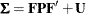whereis the factor loading matrix,is a symmetric matrix for factor correlations, andis a diagonal matrix of error variances.

If the mean structures are also analyzed, the model structures for the mean vector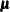of the confirmatory factor model are described in the equation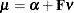where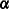is the intercept vector for the observed variables and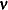is the vector for factor means. See the sections The FACTOR Model and Confirmatory Factor Analysis Models for more details about confirmatory factor models.

The FACTOR statement is the main model specification statement for the confirmatory factor model. The specifications in the FACTOR statement concern the factor loading pattern in thematrix. More details follow after a brief description of the subsidiary model specification statements: PVAR, COV, and MEAN.

By default, the factor variance parameters in the diagonal of matrixand the error variances in the diagonal of matrixare free parameters in the confirmatory factor model. However, you can override these default parameters by specifying them explicitly in the PVAR statement. For example, in some confirmatory factor models, you might want to set some of these variances to fixed constants, or you might want to set equality constraints by using the same parameter name at different parameter locations in your model.

By default, factor covariances, which are the off-diagonal elements of matrix, are free parameters in the confirmatory factor model. However, you can override these default covariance parameters by specifying them explicitly in the COV statement. Note that you cannot use the COV statement to specify the error covariances—they are always fixed zeros in the confirmatory factor analysis model.

By default, all factor means are fixed zeros and all intercepts are free parameters if the mean structures are analyzed. You can override these defaults by explicitly specifying the means of the factors in vectorand the intercepts of the manifest variables in vectorin the MEAN statement.

Because the default parameterization of the confirmatory FACTOR model already covers most commonly used parameters in matrices,,, and, the specifications in the PVAR, COV, and MEAN statements are secondary to the specifications in the FACTOR statement, which specifies the factor pattern of thematrix. The following example statement introduces the syntax of the confirmatory FACTOR statement. Suppose that there are nine manifest variables V1V9 in your sample and you want to fit a model with four factors, as shown in the following FACTOR statement:

factor
g_factor   ===>   V1-V9 ,
factor_a   ===>   V1-V3 ,
factor_b   ===>   V4-V6 ,
factor_c   ===>   V7-V9 ;


In this factor model, you assume a general factor g_factor and three group-factors: factor_a, factor_b, and factor_c. The general factor g_factor is related to all manifest variables in the sample, while each group-factor is related only to three manifest variables. This example fits the following pattern of factor pattern of:

            g_factor   factor_a   factor_b   factor_c

V1         x         x
V2         x         x
V3         x         x
V4         x                    x
V5         x                    x
V6         x                    x
V7         x                               x
V8         x                               x
V9         x                               x


where an x represents an unnamed free parameter and all other cells that are blank are fixed zeros. For each of these unnamed parameters, PROC CALIS generates a parameter name with the _Parm prefix and appended with a unique integer (for example, _Parm1, _Parm2 and so on).

An unnamed free parameter is only one of the following five types of parameters (parameter-spec) you can specify at the end of each factor-variables-relation:

• an unnamed free parameter

• an initial value

• a fixed value

• a free parameter with a name provided

• a free parameter with a name and initial value provided

To illustrate these different types of parameter specifications, consider the following factor pattern for:

            g_factor   factor_a   factor_b   factor_c



where an x represents an unnamed free parameter, a constant 1 represents a fixed value, and each name in a cell represents a name for a free parameter. You can specify this factor pattern by using the following FACTOR statement:

factor
factor_a   ===>   V1-V3    = 1. (.7  .8),
factor_c   ===>   V7-V9    = 1. 2*load_c ;


In the first entry of the FACTOR statement, you specify that the loadings of V1V9 on g_factor are free parameters g_load1g_load9 with all given an initial estimate of 0.6. The syntax 9*0.6 means that 0.6 is repeated nine times. Because they are enclosed in a pair parentheses, all these values are treated as initial estimates, but not fixed values.

The second entry of the FACTOR statement can be split into the following specification:

   factor_a   ===>   V1    = 1.  ,
factor_a   ===>   V2    = (.7),
factor_a   ===>   V3    = (.8),


This means that the first loading is a fixed value of 1, while the other loadings are unnamed free parameters with initial estimates 0.7 and 0.8, respectively. For each of these unnamed parameters with initial values, PROC CALIS also generates a parameter name with the _Parm prefix and appended with a unique integer.

The third entry of the FACTOR statement can be split into the following specification:

   factor_b   ===>   V4    = 1.  ,
factor_b   ===>   V5    = load_a (.9),


This means that the first loading is a fixed value of 1, the second loading is a free parameter named load_a with an initial estimate of 0.9, and the third loading is a free parameter named load_b without an initial estimate. PROC CALIS generates the initial value for this free parameter.

The fourth entry of the FACTOR statement states that the first loading is a fixed 1 and the remaining two loadings are free parameters named load_c. No initial estimate is given. But because the two loadings have the same parameter name, they are constrained to be equal in the estimation.

Notice that an initial value that follows after a parameter name is associated with the free parameter. For example, in the third entry of the FACTOR statement, the specification (.9) after load_a is interpreted as the initial value for the parameter load_a, but not as the initial estimate for the next loading for V6.

However, if you indeed want to specify that load_a is a free parameter without an initial value and (0.9) is an initial estimate for the loading for V6, you can use a null initial value specification for the parameter load_a, as shown in the following specification:

   factor_b   ===>   V4-V6    = 1. load_a() (.9),


This way 0.9 becomes the initial estimate of the loading for V6. Because a parameter list with mixed parameter types might be confusing, you can split the specification into separate entries to remove ambiguities. For example, you can use the following equivalent specification:

   factor_b   ===>   V4    = 1.,
factor_b   ===>   V6    = (.9),


#### Shorter and Longer Parameter Lists

If you provide fewer parameters than the number of loadings that are specified in the corresponding factor-variable-relation, all the remaining parameters are treated as unnamed free parameters. For example, the following specification assigns a fixed value of 1.0 to the first loading, while treating the remaining two loadings as unnamed free parameters:

factor
factor_a   ===>   V1-V3    = 1.;


This specification is equivalent to the following specification:

factor
factor_a   ===>   V1     = 1.,
factor_a   ===>   V2 V3      ;


If you intend to fill up all values with the last parameter specification in the list, you can use the continuation syntax [...], [..], or [.], as shown in the following example:

factor
g_factor   ===>   V1-V30    = 1.  (.5) [...];


This means that the loading of V1 on g_factor is a fixed value of 1.0, while the remaining 29 loadings are unnamed free parameters with all given an initial estimate of 0.5.

However, you must be careful not to provide too many parameters. For example, the following specification results in an error:

factor


The parameter list has six parameters for three loadings. Parameters after load3 are excessive.

#### Default Parameters

It is important to understand the default parameters in the FACTOR model. First, if you know which parameters are default free parameters, you can make your specification more efficient by omitting the specifications of those parameters that can be set by default. For example, because all error variances in the confirmatory FACTOR model are free parameters by default, you do not need to specify them with the PVAR statement if these error variances are not constrained. Second, if you know which parameters are default free parameters, you can specify your model accurately. For example, because all factor variance and covariances in the confirmatory FACTOR model are free parameters by default, you must use the COV statement to restrict the covariances among the factors if you want to fit an orthogonal factor model. See the section Default Parameters in the FACTOR Model for details about the default parameters of the FACTOR model.

#### Modifying a FACTOR Model from a Reference Model

This section assumes that you use a REFMODEL statement within the scope of a MODEL statement and that the reference model (or base model) is a factor model, either exploratory or confirmatory. The reference model is called the old model, and the model that refers to the old model is called the new model. If the new model is not intended to be an exact copy of the old FACTOR model, you can use the extended FACTOR modeling language described in this section to make modifications from the old model before transferring the specifications to the new model.

Using the REFMODEL statement for defining new factor models is not recommended in the following cases:

• If your old model is an exploratory factor analysis model, then specification by using the FACTOR modeling language in the new model replaces the old model completely. In this case, the use of the REFMODEL statement is superfluous and should be avoided.

• If your old model is a confirmatory factor analysis model, then specification of an exploratory factor model by using the FACTOR statement in the new model also replaces the old model completely. Again, the use of the REFMODEL statement is superfluous and should be avoided.

The nontrivial case where you might find the REFMODEL statement useful is when you modify an old confirmatory factor model to form a new confirmatory factor model. This nontrivial case is the focus of discussion in the remaining of the section.

The extended FACTOR modeling language for modifying model specification bears the same syntax as that of the ordinary FACTOR modeling language (see the section Confirmatory Factor Analysis). The syntax is:

 FACTOR  factor-variable-relation ;
 PVAR  partial-variance-parameters ;
 COV  covariance-parameters ;
 MEAN  mean-parameters ;

The new model is formed by integrating with the old model in the following ways:

Duplication:

If you do not specify in the new model a parameter location that exists in the old model, the old parameter specification is duplicated in the new model.

If you specify in the new model a parameter location that does not exist in the old model, the new parameter specification is added in the new model.

Deletion:

If you specify in the new model a parameter location that also exists in the old model and the new parameter is denoted by the missing value '.', the old parameter specification is not copied into the new model.

Replacement:

If you specify in the new model a parameter location that also exists in the old model and the new parameter is not denoted by the missing value '.', the new parameter specification replaces the old one in the new model.

For example, consider the following two-group analysis:

proc calis;
group 1 / data=d1;
group 2 / data=d2;
model 1 / group=1;
factor
cov
F1 F2 = c12,
F2 F3 = c23;
pvar
F1-F3 = c1-c3,
V1-V9 = ev1-ev9;
model 2 / group=2;
refmodel 1;
factor

In this specification, you specify Model 2 by referring to Model 1 in the REFMODEL statement; Model 2 is the new model which refers to the old model, Model 1. Because the PVAR statement is not used in new model, all variance and partial variance parameter specifications in the PVAR statement of the old model are duplicated in the new model. The covariance parameter c23 for covariance between F2 and F3 in the COV statement of the old model is also duplicated in the new model. Similarly, loading parameters load1load6 for some specific factor matrix locations are duplicated from the old model to the new model.
The new model has an additional parameter specification that the old model does not have. In the COV statement of the new model, covariance parameter c13 for the covariance between F1 and F3 is added.
In the same statement, the covariance between F1 and F2 is denoted by the missing value '.'. The missing value indicates that this parameter location in the old model should not be included in the new model. The consequence of this deletion from the old model is that the covariance between F1 and F2 is a fixed zero in the new model.
Finally, the three new loading specifications in the FACTOR statement of the new model replace the fixed ones in the old model. They are now free parameters loada, loadb, and loadc in the new model.